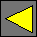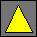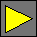July 2000 Draft JavaScript 2.0 Libraries Operator OverloadingWednesday, February 16, 2000

## Overview

Operator overloading is useful to implement Spice-style units without having to add units to the core of the JavaScript 2.0 language. Operator overloading is done via an optional library that, when imported, exposes several additional functions and methods. This library is analogous to the internationalization library in that it does not have to be present on all implementations of JavaScript 2.0; implementations without this library do not support operator overloading.

## Interface

To override operators, import package `Operators`, version `1`.

### Unary Operators

After importing package `Operators`, the following methods become available on all objects. Override these to override the behavior of unary operators.

Method Operator
`Operator::plus()` `+`expr
`Operator::minus()` `-`expr
`Operator::bitwiseNot()` `~`expr
`Operator::preIncrement()` `++`expr
`Operator::postIncrement()` expr`++`
`Operator::preDecrement()` `--`expr
`Operator::postDecrement()` expr`--`
`Operator::call(a1,` ...`,` `an)` expr`(`a1`,` ...`,` an`)`
`Operator::construct(a1,` ...`,` `an)`   `new` expr`(`a1`,` ...`,` an`)`
`Operator::lookup(a1,` ...`,` `an)` expr`[`a1`,` ...`,` an`]`
`Operator::toBoolean():Boolean` `if (`expr`)` ..., etc.

The `preIncrement`, `postIncrement`, `preDecrement`, and `postDecrement` operators should return a two-element array; the first element should be the result of the operator, while the second should be a new value to be stored as the new value of the incremented or decremented variable. The other operators should return a result of the expression.

The `call`, `construct`, and `lookup` operators also take argument lists. If desired, these argument lists can include optional or `...` arguments.

The `!`, `||`, `^^`, `&&`, and `? :` operators cannot be overridden directly, but they are affected by any redefinition of `toBoolean`.

### Binary Operators

After importing package `Operators`, the following global functions become available to override binary operators:

Function Operator
`defineAdd(T1:Type, T2:Type, F:Function)` `+`
`defineSubtract(T1:Type, T2:Type, F:Function)` `-`
`defineMultiply(T1:Type, T2:Type, F:Function)` `*`
`defineDivide(T1:Type, T2:Type, F:Function)` `/`
`defineRemainder(T1:Type, T2:Type, F:Function)` `%`
`defineLeftShift(T1:Type, T2:Type, F:Function)` `<<`
`defineRightShift(T1:Type, T2:Type, F:Function)` `>>`
`defineLogicalRightShift(T1:Type, T2:Type, F:Function)` `>>>`
`defineBitwiseOr(T1:Type, T2:Type, F:Function)` `|`
`defineBitwiseXor(T1:Type, T2:Type, F:Function)` `^`
`defineBitwiseAnd(T1:Type, T2:Type, F:Function)` `&`
`defineLess(T1:Type, T2:Type, F:Function)` `<`
`defineLessOrEqual(T1:Type, T2:Type, F:Function)`    `<=`
`defineEqual(T1:Type, T2:Type, F:Function)` `==`
`defineIdentical(T1:Type, T2:Type, F:Function)` `===`

Each of these functions defines the meaning of an operator for the case where its first operand has type `T1` and the second operand has type `T2`. At least one of these types must be a class defined in the current package. `F` is a function that takes two arguments (of type `T1` and `T2`) and produces the operator's result. The function `F` used to override the `<`, `<=`, `==`, and `===` operators should return a `Boolean`; the results of the other operators are unrestricted.

When one of the operators op above is invoked in an expression a op b, the most specific definition of op that matches a and b is invoked. A definition of op for types t1 and t2 matches if the value of a is a member of t1 and the value of b is a member of t2. A definition of op for types t1 and t2 is most specific if it matches and if every other matching definition of op for types s1 and s2 satisfies t1 s1 and t2 s2. If there is no most specific matching definition of op then an error occurs.

After an operator is defined for a particular pair of types `T1` and `T2` it cannot be changed. A static implementation may restrict calls to the above `define`... functions to occur only in compiler blocks.

The `>`, `>=`, `!=`, and `!==` operators cannot be overridden directly; instead, they are defined in terms of `<`, `<=`, `==`, and `===`:

Expression   Definition
a `>` b b `<` a
a `>=` b b `<=` a
a `!=` b `!(`a `==` b`)`
a `!==` b `!(`a `===` b`)`

 Waldemar Horwat Last modified Wednesday, February 16, 2000